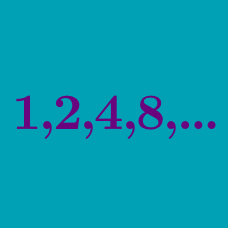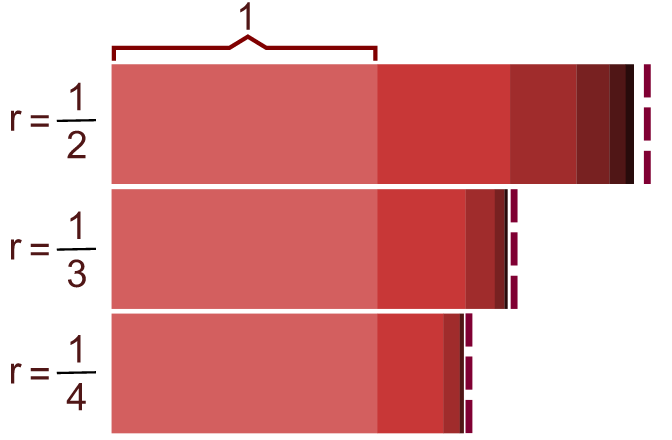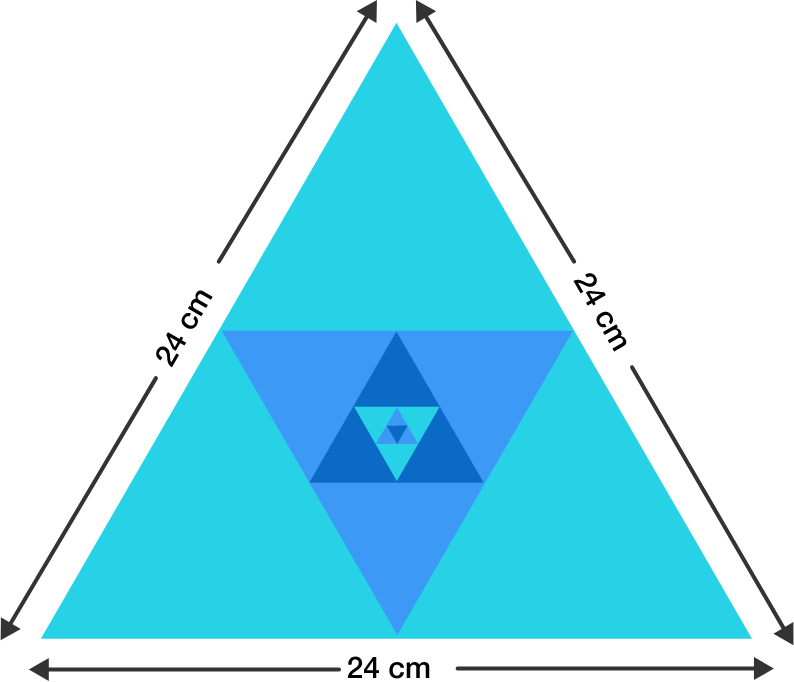Algebra

# Geometric Progressions: Level 1 Challenges$1+\frac{1}{3}+\frac{1}{9}+\frac{1}{27}+\ldots$

What is the value of the above series?

###### Image Credit: Wikimedia Geometric ProgressionOne side of an equilateral triangle is 24 cm. The midpoints of its sides are joined to form another triangle whose midpoints, in turn, are joined to form still another triangle. This process continues indefinitely.

Find the sum of the perimeters of all these triangles that are defined above.

$\left(\dfrac1{2^2}+\dfrac1{3^2}+\dfrac1{4^2}+\ldots\right) + \\ \hspace{1cm}\left(\dfrac1{2^3}+\dfrac1{3^3}+\dfrac1{4^3} + \ldots\right) + \\ \hspace{2.5cm}\left(\dfrac1{2^4}+\dfrac1{3^4}+\dfrac1{4^4}+\ldots\right)+\cdots$

What is the value of the series above?

The sum of the first 2011 terms of the geometric sequence is 200.

The sum of the first 4022 terms of the same geometric sequence is 380.

Find the sum of the first 6033 terms of this geometric sequence.I have a pint of orange juice. I drink half of it and then give it to you. You drink half of what's remaining, and then pass it back to me. I drink half of what's remaining and then give it to you.

If this process continues forever, how much of the pint will I drink?

×